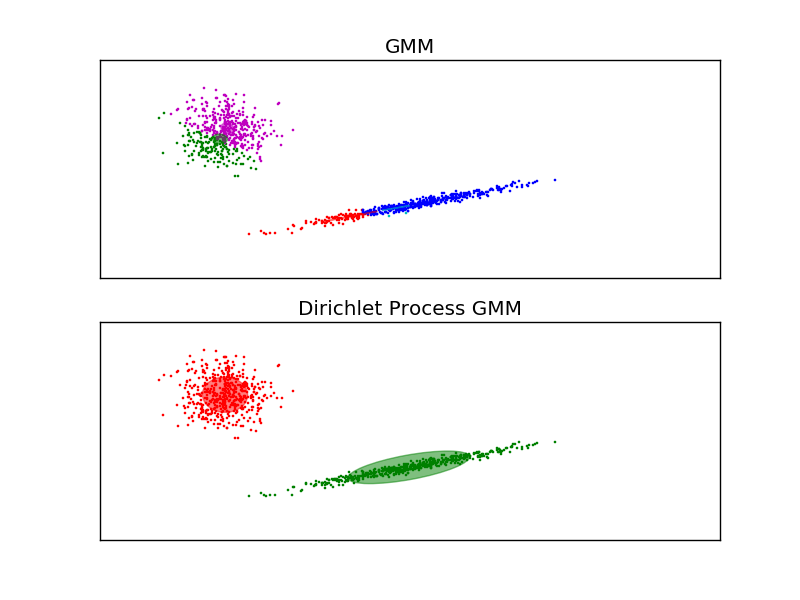# Gaussian Mixture Model Ellipsoids¶

Plot the confidence ellipsoids of a mixture of two Gaussians with EM and variational Dirichlet process.

Both models have access to five components with which to fit the data. Note that the EM model will necessarily use all five components while the DP model will effectively only use as many as are needed for a good fit. This is a property of the Dirichlet Process prior. Here we can see that the EM model splits some components arbitrarily, because it is trying to fit too many components, while the Dirichlet Process model adapts it number of state automatically.

This example doesn’t show it, as we’re in a low-dimensional space, but another advantage of the Dirichlet process model is that it can fit full covariance matrices effectively even when there are less examples per cluster than there are dimensions in the data, due to regularization properties of the inference algorithm.Python source code: `plot_gmm.py`

```import itertools

import numpy as np
from scipy import linalg
import matplotlib.pyplot as plt
import matplotlib as mpl

from sklearn import mixture

# Number of samples per component
n_samples = 500

# Generate random sample, two components
np.random.seed(0)
C = np.array([[0., -0.1], [1.7, .4]])
X = np.r_[np.dot(np.random.randn(n_samples, 2), C),
.7 * np.random.randn(n_samples, 2) + np.array([-6, 3])]

# Fit a mixture of Gaussians with EM using five components
gmm = mixture.GMM(n_components=5, covariance_type='full')
gmm.fit(X)

# Fit a Dirichlet process mixture of Gaussians using five components
dpgmm = mixture.DPGMM(n_components=5, covariance_type='full')
dpgmm.fit(X)

color_iter = itertools.cycle(['r', 'g', 'b', 'c', 'm'])

for i, (clf, title) in enumerate([(gmm, 'GMM'),
(dpgmm, 'Dirichlet Process GMM')]):
splot = plt.subplot(2, 1, 1 + i)
Y_ = clf.predict(X)
for i, (mean, covar, color) in enumerate(zip(
clf.means_, clf._get_covars(), color_iter)):
v, w = linalg.eigh(covar)
u = w / linalg.norm(w)
# as the DP will not use every component it has access to
# unless it needs it, we shouldn't plot the redundant
# components.
if not np.any(Y_ == i):
continue
plt.scatter(X[Y_ == i, 0], X[Y_ == i, 1], .8, color=color)

# Plot an ellipse to show the Gaussian component
angle = np.arctan(u / u)
angle = 180 * angle / np.pi  # convert to degrees
ell = mpl.patches.Ellipse(mean, v, v, 180 + angle, color=color)
ell.set_clip_box(splot.bbox)
ell.set_alpha(0.5)